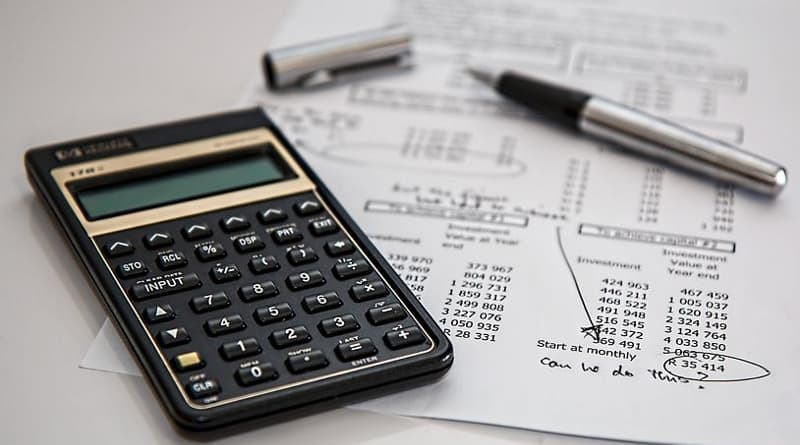# Real Estate Mathematics## Math is a language and a tool used in all areas of Real Estate to determine housing price. When it comes to calculating the price of a real estate, Metropolitan for example, it is possible to use simple math like that of addition, subtraction, multiplication and division.

Out of all the real estate formulas, the most basic Real Estate formula you need to know is the T-Method. Because as you go through many Real Estate problems, you will find out that they are just another version of the T-Method. So it is necessary that you thoroughly understand the T-Method Real Estate math formula.

The T-Method essentially shows the relationship between Total, Part and Rate and is visualized by drawing a T.

Part

 Total Rate (%)

The top of the T indicates we need to divide and the vertical line of the T shows that we need to multiply. Based on the T-Method we get the following formulas

 Part / Total = Rate Part / Rate = Total Total * Rate = Part

Below are a few numerical examples to determine the Part, Total or Rate of Metropolitan Real Estate that is up for sale:

• Example 1: Let us say for example that Metropolitan Real Estate is selling a house for birr 4,000,000.00 and the commission rate is 7%. What will be the commission amount?

In this example, the total is birr 4,000,000.00 and the rate is 6%. So, we can use the T-Method to see that the actual commission is calculated by birr 4,000,000.00*0.07 = birr 280,000.00

• Example 2: For this example, let us consider that Metropolitan Real Estate is selling a house for birr 4,000,000.00 and brokerage X receives birr 100,000.00 in commission. What will be the commission rate?

In this example, the total is birr 4,000,000.00 and the part (actual commission) is birr10,000.00. So, we use the T-Method to see that the commission rate is calculated by birr 100,000.00/birr 4,000,000.00=0.025 or 2.5%

• Example 3: Let us consider that brokerage X receives birr 200,000.00 in commission and the commission rate is supposedly 11%. What would be the selling price of the house?

In this example, the part (actual commission) is birr 200,000.00 and the rate is 11%. So, by using the T-Method, we see that the total selling price is calculated by birr 200,000.00/0.11= birr 1,820,000.00

When you come across a real estate math question, since most of the time two pieces of the T-Method are given, it will be your duty to find the missing piece. So when solving a real estate math problem, always try to find out the total, part or rate first.